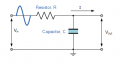Calculate component values

pinkyponky

Joined Nov 28, 2019
235
Hi,

How to calculate the RC low-pass filter components values?, the filter to be used on DC 5V before the non-inverting amplifier inputs.

Papabravo

Joined Feb 24, 2006
17,569
Hi,

How to calculate the RC low-pass filter components values?, the filter to be used on DC 5V before the non-inverting amplifier inputs.
You want an RC low pass filter. The problem is that the solution is not unique. In fact there are infinitely many solutions. The cutoff frequency is given by:

$$f_c\;=\;(2\pi RC)^{-1}$$

You can pick a convenient value for C and compute an approximate value for R. You can also pick a convenient value for R and compute an approximate value for C. This will give you a 1-stage RC filter and it will work as long as you hook it up correctly with the input going to R and the output across the capacitor and Ground. Like this